# Whether the graph is function and if it is a function then whether it is one-to-one.### Precalculus: Mathematics for Calcu...

6th Edition
Stewart + 5 others
Publisher: Cengage Learning
ISBN: 9780840068071### Precalculus: Mathematics for Calcu...

6th Edition
Stewart + 5 others
Publisher: Cengage Learning
ISBN: 9780840068071

#### Solutions

Chapter 2, Problem 1T

(a)

To determine

## To describe: Whether the graph is function and if it is a function then whether it is one-to-one.

Expert Solution

### Explanation of Solution

The given graph is,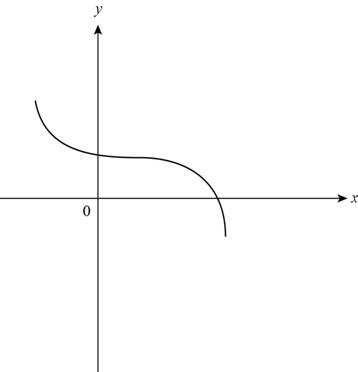Figure (1)

Use vertical line test to find whether the given graph in Figure (1) is function or not.

Vertical line test states that, if a vertical line intersects the graph at more than one point than the graph is not a function.

Use horizontal line test to find whether the function is one-to-one.

Horizontal line states that if a horizontal line intersects the function more than once than the function is not one-to-one.

Draw a vertical line and horizontal line as shown below,Figure (2)

From Figure (2) observe that line a intersect the graph at only one point p and line b intersect the graph of function at one point q.

The vertical line intersects at one point and horizontal line also intersects at one point.

Thus, the given graph is a function and it is one-to-one.

(b)

To determine

Expert Solution

### Explanation of Solution

The given graph is,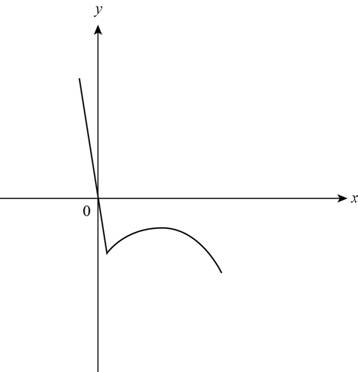Figure (3)

Use vertical line test to find whether the given graph in Figure (1) is function or not.

Vertical line test states that, if a vertical line intersects the graph at more than one point than the graph is not a function.

Use horizontal line test to find whether the function is one-to-one.

Horizontal line states that if a horizontal line intersects the function more than once than the function is not one-to-one.

Draw a vertical line and a horizontal line as shown below,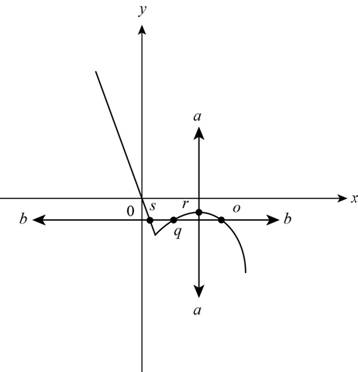Figure (4)

From Figure (4) it can be observe that line a intersect at only one point r and line b intersect the function at three points s, q and p.

Vertical line intersects at only one point. So the graph is a function.

Horizontal line intersects at more than one point. So, the function is not one-to-one.

Thus, the given graph is a function but not one-to-one.

(c)

To determine

Expert Solution

### Explanation of Solution

The given graph is,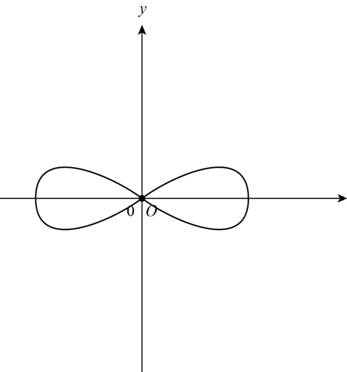Figure (5)

Use vertical line test to find whether the given graph in Figure (5) is function or not.

Vertical line test states that, if a vertical line intersects the graph at more than one point than the graph is not a function.

Use horizontal line test to find whether the function is one-to-one.

Horizontal line states that if a horizontal line intersects the function more than once than the function is not one-to-one.

Draw a vertical line and a horizontal line as shown below,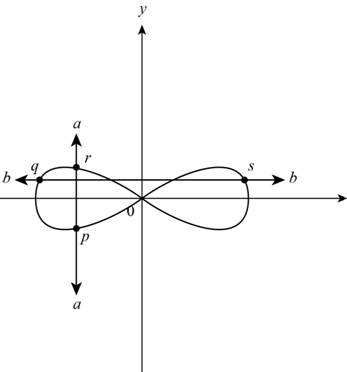Figure (6)

From Figure (2) it can be observe that vertical line a intersect at points p and r. The horizontal line b intersect the function at points q and s.

So, vertical line intersects at more than one point and horizontal line also intersects at more than one point.

Thus, the given graph is not a function and not one-to-one.

(d)

To determine

Expert Solution

### Explanation of Solution

The given graph is,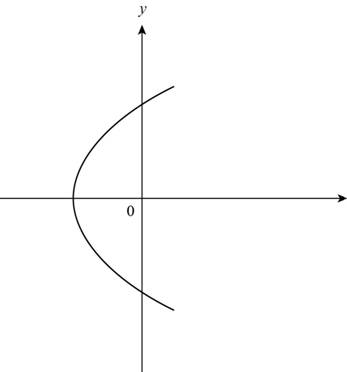Figure (7)

Use vertical line test to find whether the given graph in Figure (7) is function or not.

Vertical line test states that, if a vertical line intersects the graph at more than one point than the graph is not a function.

Use horizontal line test to find whether the function is one-to-one.

Horizontal line states that if a horizontal line intersects the function more than once than the function is not one-to-one.

Draw a vertical line and horizontal line as shown below,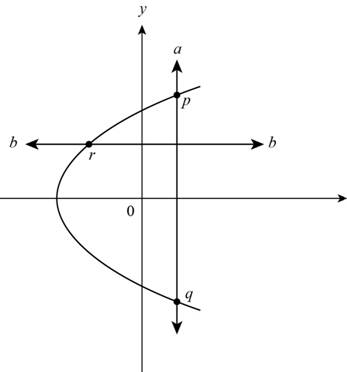Figure (8)

From Figure (8) it can be observe that vertical line a intersects at two points p and q. The horizontal line b intersects the function at only one point r.

Vertical line intersects the graph at more than one point and horizontal line intersects at only one point.

Thus, the given graph is a not a function but one-to-one.

### Have a homework question?

Subscribe to bartleby learn! Ask subject matter experts 30 homework questions each month. Plus, you’ll have access to millions of step-by-step textbook answers!